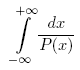ENG  RUS Timus Online JudgeOnline Judge
Задачи
Авторы
Соревнования
О системе
Часто задаваемые вопросы
Новости сайта
Форум
Ссылки
Архив задач
Отправить на проверку
Состояние проверки
Руководство
Регистрация
Исправить данные
Рейтинг авторов
Текущее соревнование
Расписание
Прошедшие соревнования
Правила

## SPbSU ITMO contest. Petrozavodsk training camp. Winter 2008

Соревнование завершено

## D. Definite Integral

Ограничение времени: 1.0 секунды
Ограничение памяти: 64 МБ
Your task is to evaluate the following definite integral:where P(x) = a4 · x4 + a3 · x3 + a2 · x2 + a1 · x + a0. P(x) has no real roots and GCD(P(x), P'(x)) = const.

### Исходные данные

The input contains five integers: a0, a1, a2, a3 and a4 separated by whitespace. Each of these numbers does not exceed 106 by absolute value, a4 ≠ 0.

### Результат

Output the value of the integral. Assume that the exact value is A and your answer is B. Your answer will be considered correct if and only if at least one of the following statements is true:
• A − 10−9BA + 10−9
• A · (1 − 10−9) ≤ BA · (1 + 10−9)

### Пример

исходные данныерезультат
`16 0 0 0 1`
```0.2776801836
```
Источник задачи: SPbSU ITMO contest. Petrozavodsk training camp. Winter 2008.
Чтобы отправить решение этой задачи на проверку перейдите в Архив задач: 1621. Definite Integral